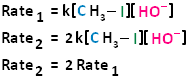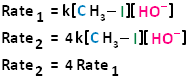# Potential Energy Diagram

$$\newcommand{\vecs}{\overset { \rightharpoonup} {\mathbf{#1}} }$$ $$\newcommand{\vecd}{\overset{-\!-\!\rightharpoonup}{\vphantom{a}\smash {#1}}}$$$$\newcommand{\id}{\mathrm{id}}$$ $$\newcommand{\Span}{\mathrm{span}}$$ $$\newcommand{\kernel}{\mathrm{null}\,}$$ $$\newcommand{\range}{\mathrm{range}\,}$$ $$\newcommand{\RealPart}{\mathrm{Re}}$$ $$\newcommand{\ImaginaryPart}{\mathrm{Im}}$$ $$\newcommand{\Argument}{\mathrm{Arg}}$$ $$\newcommand{\norm}{\| #1 \|}$$ $$\newcommand{\inner}{\langle #1, #2 \rangle}$$ $$\newcommand{\Span}{\mathrm{span}}$$ $$\newcommand{\id}{\mathrm{id}}$$ $$\newcommand{\Span}{\mathrm{span}}$$ $$\newcommand{\kernel}{\mathrm{null}\,}$$ $$\newcommand{\range}{\mathrm{range}\,}$$ $$\newcommand{\RealPart}{\mathrm{Re}}$$ $$\newcommand{\ImaginaryPart}{\mathrm{Im}}$$ $$\newcommand{\Argument}{\mathrm{Arg}}$$ $$\newcommand{\norm}{\| #1 \|}$$ $$\newcommand{\inner}{\langle #1, #2 \rangle}$$ $$\newcommand{\Span}{\mathrm{span}}$$$$\newcommand{\AA}{\unicode[.8,0]{x212B}}$$

We will be contrasting about two types of nucleophilic substitution reactions. One type is referred to as unimolecular nucleophilic substitution (SN1), whereby the rate determining step is unimolecular and bimolecular nucleophilic substitution (SN2), whereby the rate determining step is bimolecular. We will begin our discussion with SN2 reactions, and discuss SN1 reactions elsewhere.

## Biomolecular Nucleophilic Substitution Reactions and Kinetics

In the term SN2, the S stands for substitution, the N stands for nucleophilic, and the number two stands for bimolecular, meaning there are two molecules involved in the rate determining step. The rate of bimolecular nucleophilic substitution reactions depends on the concentration of both the haloalkane and the nucleophile. To understand how the rate depends on the concentrations of both the haloalkane and the nucleophile, let us look at the following example. The hydroxide ion is the nucleophile and methyl iodide is the haloalkane.If we were to double the concentration of either the haloalkane or the nucleophile, we can see that the rate of the reaction would proceed twice as fast as the initial rate.If we were to double the concentration of both the haloalkane and the nucleophile, we can see that the rate of the reaction would proceed four times as fast as the initial rate.The bimolecular nucleophilic substitution reaction follows second-order kinetics; that is, the rate of the reaction depends on the concentration of two first-order reactants. In the case of bimolecular nucleophilic substitution, these two reactants are the haloalkane and the nucleophile. For further clarification on reaction kinetics, the following links may facilitate your understanding of rate laws, rate constants, and second-order kinetics:

## Bimolecular Nucleophilic Substitution Reactions Are Concerted

Bimolecular nucleophilic substitution (SN2) reactions are concerted, meaning they are a one step process. This means that the process whereby the nucleophile attacks and the leaving group leaves is simultaneous. Hence, the bond-making between the nucleophile and the electrophilic carbon occurs at the same time as the bond-breaking between the electophilic carbon and the halogen.

The potential energy diagram for an SN2 reaction is shown below. Upon nucleophilic attack, a single transition state is formed. A transition state, unlike a reaction intermediate, is a very short-lived species that cannot be isolated or directly observed. Again, this is a single-step, concerted process with the occurrence of a single transition state.## Contributors• Racheal Curtis

Potential Energy Diagram is shared under a CC BY-NC-SA 4.0 license and was authored, remixed, and/or curated by LibreTexts.